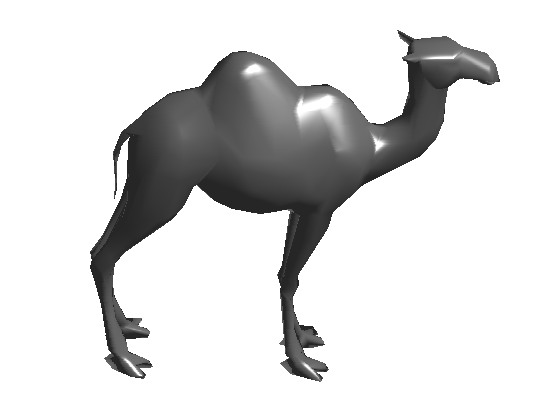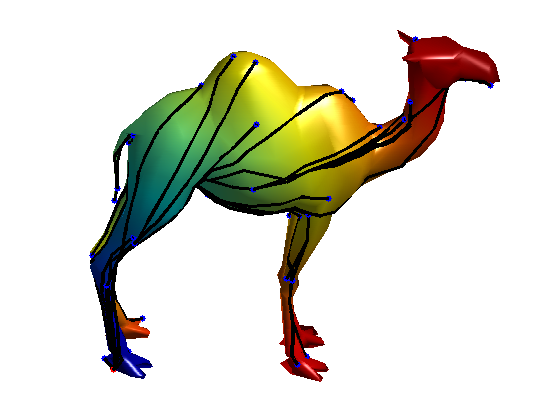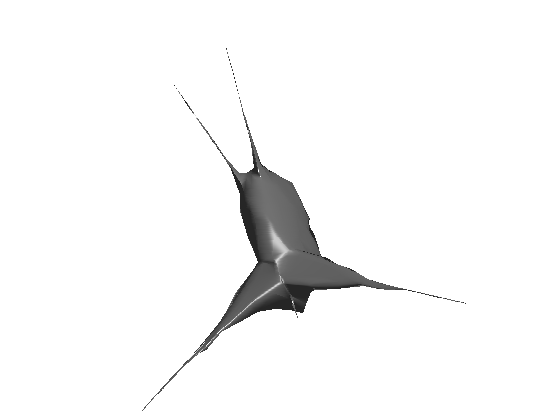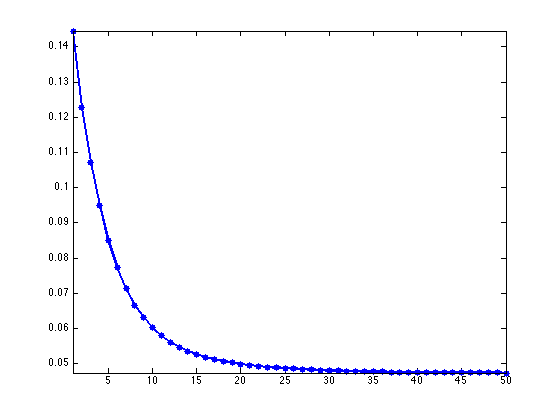$\newcommand{\NN}{\mathbb{N}} \newcommand{\CC}{\mathbb{C}} \newcommand{\GG}{\mathbb{G}} \newcommand{\LL}{\mathbb{L}} \newcommand{\PP}{\mathbb{P}} \newcommand{\QQ}{\mathbb{Q}} \newcommand{\RR}{\mathbb{R}} \newcommand{\VV}{\mathbb{V}} \newcommand{\ZZ}{\mathbb{Z}} \newcommand{\FF}{\mathbb{F}} \newcommand{\KK}{\mathbb{K}} \newcommand{\UU}{\mathbb{U}} \newcommand{\EE}{\mathbb{E}} \newcommand{\Aa}{\mathcal{A}} \newcommand{\Bb}{\mathcal{B}} \newcommand{\Cc}{\mathcal{C}} \newcommand{\Dd}{\mathcal{D}} \newcommand{\Ee}{\mathcal{E}} \newcommand{\Ff}{\mathcal{F}} \newcommand{\Gg}{\mathcal{G}} \newcommand{\Hh}{\mathcal{H}} \newcommand{\Ii}{\mathcal{I}} \newcommand{\Jj}{\mathcal{J}} \newcommand{\Kk}{\mathcal{K}} \newcommand{\Ll}{\mathcal{L}} \newcommand{\Mm}{\mathcal{M}} \newcommand{\Nn}{\mathcal{N}} \newcommand{\Oo}{\mathcal{O}} \newcommand{\Pp}{\mathcal{P}} \newcommand{\Qq}{\mathcal{Q}} \newcommand{\Rr}{\mathcal{R}} \newcommand{\Ss}{\mathcal{S}} \newcommand{\Tt}{\mathcal{T}} \newcommand{\Uu}{\mathcal{U}} \newcommand{\Vv}{\mathcal{V}} \newcommand{\Ww}{\mathcal{W}} \newcommand{\Xx}{\mathcal{X}} \newcommand{\Yy}{\mathcal{Y}} \newcommand{\Zz}{\mathcal{Z}} \newcommand{\al}{\alpha} \newcommand{\la}{\lambda} \newcommand{\ga}{\gamma} \newcommand{\Ga}{\Gamma} \newcommand{\La}{\Lambda} \newcommand{\Si}{\Sigma} \newcommand{\si}{\sigma} \newcommand{\be}{\beta} \newcommand{\de}{\delta} \newcommand{\De}{\Delta} \renewcommand{\phi}{\varphi} \renewcommand{\th}{\theta} \newcommand{\om}{\omega} \newcommand{\Om}{\Omega} \renewcommand{\epsilon}{\varepsilon} \newcommand{\Calpha}{\mathrm{C}^\al} \newcommand{\Cbeta}{\mathrm{C}^\be} \newcommand{\Cal}{\text{C}^\al} \newcommand{\Cdeux}{\text{C}^{2}} \newcommand{\Cun}{\text{C}^{1}} \newcommand{\Calt}{\text{C}^{#1}} \newcommand{\lun}{\ell^1} \newcommand{\ldeux}{\ell^2} \newcommand{\linf}{\ell^\infty} \newcommand{\ldeuxj}{{\ldeux_j}} \newcommand{\Lun}{\text{\upshape L}^1} \newcommand{\Ldeux}{\text{\upshape L}^2} \newcommand{\Lp}{\text{\upshape L}^p} \newcommand{\Lq}{\text{\upshape L}^q} \newcommand{\Linf}{\text{\upshape L}^\infty} \newcommand{\lzero}{\ell^0} \newcommand{\lp}{\ell^p} \renewcommand{\d}{\ins{d}} \newcommand{\Grad}{\text{Grad}} \newcommand{\grad}{\text{grad}} \renewcommand{\div}{\text{div}} \newcommand{\diag}{\text{diag}} \newcommand{\pd}{ \frac{ \partial #1}{\partial #2} } \newcommand{\pdd}{ \frac{ \partial^2 #1}{\partial #2^2} } \newcommand{\dotp}{\langle #1,\,#2\rangle} \newcommand{\norm}{|\!| #1 |\!|} \newcommand{\normi}{\norm{#1}_{\infty}} \newcommand{\normu}{\norm{#1}_{1}} \newcommand{\normz}{\norm{#1}_{0}} \newcommand{\abs}{\vert #1 \vert} \newcommand{\argmin}{\text{argmin}} \newcommand{\argmax}{\text{argmax}} \newcommand{\uargmin}{\underset{#1}{\argmin}\;} \newcommand{\uargmax}{\underset{#1}{\argmax}\;} \newcommand{\umin}{\underset{#1}{\min}\;} \newcommand{\umax}{\underset{#1}{\max}\;} \newcommand{\pa}{\left( #1 \right)} \newcommand{\choice}{ \left\{ \begin{array}{l} #1 \end{array} \right. } \newcommand{\enscond}{ \left\{ #1 \;:\; #2 \right\} } \newcommand{\qandq}{ \quad \text{and} \quad } \newcommand{\qqandqq}{ \qquad \text{and} \qquad } \newcommand{\qifq}{ \quad \text{if} \quad } \newcommand{\qqifqq}{ \qquad \text{if} \qquad } \newcommand{\qwhereq}{ \quad \text{where} \quad } \newcommand{\qqwhereqq}{ \qquad \text{where} \qquad } \newcommand{\qwithq}{ \quad \text{with} \quad } \newcommand{\qqwithqq}{ \qquad \text{with} \qquad } \newcommand{\qforq}{ \quad \text{for} \quad } \newcommand{\qqforqq}{ \qquad \text{for} \qquad } \newcommand{\qqsinceqq}{ \qquad \text{since} \qquad } \newcommand{\qsinceq}{ \quad \text{since} \quad } \newcommand{\qarrq}{\quad\Longrightarrow\quad} \newcommand{\qqarrqq}{\quad\Longrightarrow\quad} \newcommand{\qiffq}{\quad\Longleftrightarrow\quad} \newcommand{\qqiffqq}{\qquad\Longleftrightarrow\qquad} \newcommand{\qsubjq}{ \quad \text{subject to} \quad } \newcommand{\qqsubjqq}{ \qquad \text{subject to} \qquad }$

Geodesic Bending Invariants for Surfaces

# Geodesic Bending Invariants for Surfaces

This tour explores the computation of bending invariants of surfaces.

## Installing toolboxes and setting up the path.

You need to download the following files: signal toolbox, general toolbox and graph toolbox.

You need to unzip these toolboxes in your working directory, so that you have toolbox_signal, toolbox_general and toolbox_graph in your directory.

For Scilab user: you must replace the Matlab comment '%' by its Scilab counterpart '//'.

Recommandation: You should create a text file named for instance numericaltour.sce (in Scilab) or numericaltour.m (in Matlab) to write all the Scilab/Matlab command you want to execute. Then, simply run exec('numericaltour.sce'); (in Scilab) or numericaltour; (in Matlab) to run the commands.

Execute this line only if you are using Matlab.

getd = @(p)path(p,path); % scilab users must *not* execute this


Then you can add the toolboxes to the path.

getd('toolbox_signal/');
getd('toolbox_general/');
getd('toolbox_graph/');


## Bending Invariants

Bending invariants replace the position of the vertices in a shape $$\Ss$$ (2-D or 3-D) by new positions that are insensitive to isometric deformation of the shape. This defines a bending invariant signature that can be used for surface matching.

Bending invariant were introduced in [EladKim03]. A related method was developped for brain flattening in [SchwShWolf89]. This method is related to the Isomap algorithm for manifold learning [TenSolvLang03].

We assume that $$Ss$$ has some Riemannian metric, for instance coming from the embedding of a surface in 3-D Euclidian space, or by restriction of the Euclian 2-D space to a 2-D sub-domain (planar shape). One thus can compute the geodesic distance $$d(x,x')$$ between points $$x,x' \in \Ss$$.

The bending invariant $$\tilde \Ss$$ of $$\Ss$$ is defined as the set of points $$Y = (y_i)_j \subset \RR^d$$ that are optimized so that the Euclidean distance between points in $$Y$$ matches as closely the geodesic distance between points in $$X$$, i.e. $\forall i, j, \quad \norm{y_i-y_j} \approx d(x_i,x_j)$

Multi-dimensional scaling (MDS) is a class of method that aims at computing such a set of points $$Y \in \RR^{d \times N}$$ in $$\RR^d$$ such that $\forall i, j, \quad \norm{y_i-y_j} \approx \de_{i,j}$ where $$\de \in \RR^{N \times N}$$ is a input data matrix. For a detailed overview of MDS, we refer to the book [BorgGroe97]

In this tour, we apply two specific MDS algorithms (strain and stress minimization) with input $$\de_{i,j} = d(x_i,x_j)$$.

## 3-D Surfaces and Geodesic Distances

We consider here a syrface $$\Ss \subset \RR^3$$.

Load a mesh of $$N$$ vertices that discretizes this surfaces.

name = 'camel';
options.name = name;
N = size(V,2);


Display it.

clf;
plot_mesh(V,F, options);The geodesic distance map $$U(x) = d(x,x_i)$$ to a starting point $$x_i$$ can be computed in $$O(N \log(N))$$ operations on a mesh of $$N$$ vertices using the Fast Marching algorithm.

i = 1;
U = perform_fast_marching_mesh(V, F, i);


Extract a bunch of geodesic shortest paths from $$x_i$$ to randomly selected vertices $$(x_j)_{j \in J}$$.

options.method = 'continuous';
J = randperm(N); J = J(1:50);
paths = compute_geodesic_mesh(U, V, F, J, options);


Display the distance $$U$$ on the 3-D mesh together with the geodesic paths.

clf;
plot_fast_marching_mesh(V, F, U, paths, options);The geodesic distance matrix $$\de \in \RR^{N \times N}$$ is defined as $\forall i,j=1,\ldots,N, \quad \de_{i,j} = d(x_i,x_j).$

Exercice 1: (check the solution) Compute the geodesic distance matrix $$\de$$. It is going to take some of time.

exo1;


## Bending Invariant with Strain Minimization

The goal is to compute a set of points $$Y = (y_i)_{i=1}^N$$ in $$\RR^d$$, (here we use $$d=2$$) stored in a matrix $$Y \in \RR^{d \times N}$$ such that $\forall i, j, \quad D^2(Y)_{i,j} \approx \de_{i,j}^2 \qwhereq D^2(Y)_{i,j} = \norm{y_i-y_j}^2.$

Target dimensionality $$d$$.

d = 3;


This can be achieved by minimzing a $$L^2$$ loss $\umin{Y} \norm{ D^2(Y)-\de^2 }^2 = \sum_{i<j} \abs{ \norm{y_i-y_j}^2 - \de_{i,j}^2 }^2.$

Strain minimization consider instead the following weighted $$L^2$$ loss (so-called strain) $\umin{Y \in \RR^{d \times N} } \text{Strain}(Y) = \norm{ J ( D^2(Y)-\de^2 ) J }^2$ where $$J$$ is the so-called centering matrix $J_{i,j} = \choice{ 1-1/N \qifq i=j, \\ -1/N \qifq i \neq j. }$

J = eye(N) - ones(N)/N;


Using the properties of squared-distance matrices $$D^2(Y)$$, one can show that $\norm{ J ( D^2(Y)-\de^2 ) J }^2 = \norm{ Y Y^* - K }^2 \qwhereq K = - \frac{1}{2} J \de^2 J.$

K = -1/2 * J*(delta.^2)*J;


The solution to this (non-convex) optimization problem can be computed exactly as the rows of $$Y$$ being the two leading eigenvectors of $$K$$ propery rescaled.

opt.disp = 0;
[Y, v] = eigs(K, d, 'LR', opt);
Y = Y .* repmat(sqrt(diag(v))', [N 1]);
Y = Y';


Display the bending invariant surface.

clf;
plot_mesh(Y,F, options);## Bending Invariant with Stress Minimization

The stress functional does not have geometrical meaning. An alternative MDS method directly minimizes a geometric loss, the so-called Stress $\umin{Y \in \RR^{d \times N} } \text{Stress}(Y) = \norm{ D(Y)-\de }^2 = \sum_{i<j} \abs{ \norm{y_i-y_j} - \de_{i,j} }^2.$ It is possible to find a local minimizer of this energy by various descent algorithms, as initially proposed by [Kruskal64]

Stress = @(d)sqrt( sum( abs(delta(:)-d(:)).^2 ) / N^2 );


Operator to compute the distance matrix $$D(Y)$$.

D = @(Y)sqrt( repmat(sum(Y.^2),N,1) + repmat(sum(Y.^2),N,1)' - 2*Y'*Y);


The SMACOF (Scaling by majorizing a convex function) algorithm solves at each iterations a quadratic energy, that is guaranteed to diminish the value of the Strain. It was introduced by [Leeuw77]

It computes iterates $$X^{(\ell)}$$ as $X^{(\ell+1)} = \frac{1}{N} X^{(\ell)} B(D(X^{(\ell)}))^*,$ where $B(D) = \choice{ -\frac{\de_{i,j}}{D_{i,j}} \qifq i \neq j, \\ -\sum_{k} B(D)_{i,k} \qifq i = j. }$

Initialize the positions for the algorithm.

Y = V;


Operator $$B$$.

remove_diag = @(b)b - diag(sum(b));
B = @(D1)remove_diag( -delta./max(D1,1e-10) );


Update the positions.

Y = Y * B(D(Y))' / N;


Exercice 2: (check the solution) Perform the SMACOF iterative algorithm. Save in a variable s(l) the values of Stress$$( X^{(\ell)} )$$.

exo2;Plot stress evolution during minimization.

clf;
plot(s, '.-', 'LineWidth', 2, 'MarkerSize', 20);
axis('tight');Plot the optimized invariant shape.

clf;
plot_mesh(Y,F, options);## Surface Retrieval with Bending Invariant.

One can compute a bending invariant signature for each mesh in a library of 3D surface.

Isometry-invariant retrival is then perform by matching the invariant signature.

Exercice 3: (check the solution) Implement a surface retrival algorithm based on these bending invariants.

exo3;